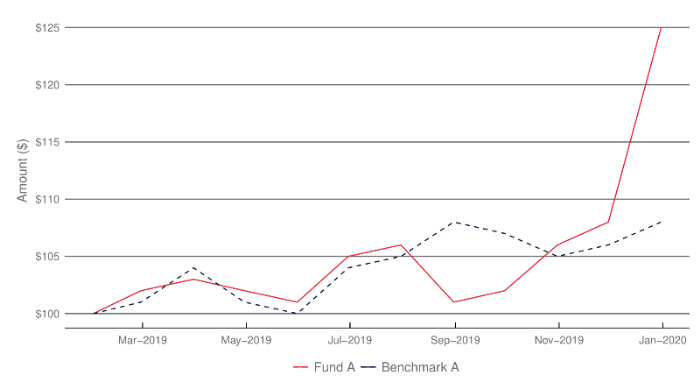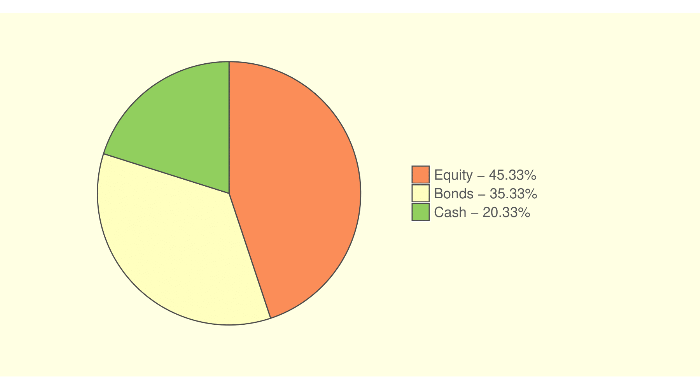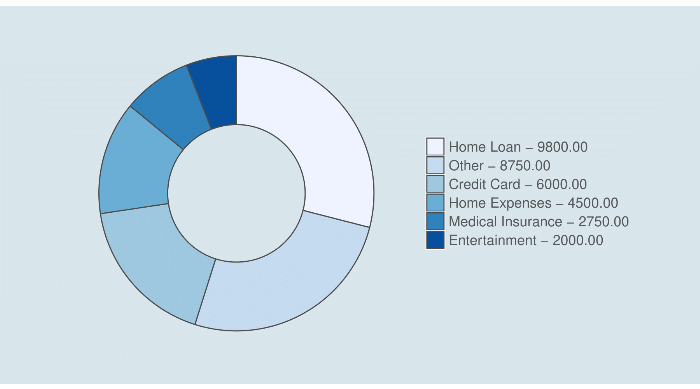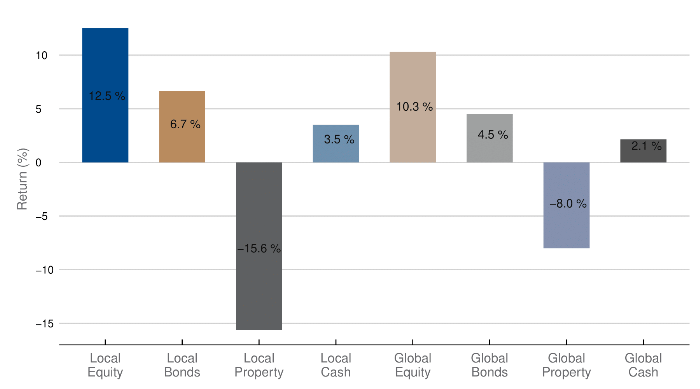Creating Charts | Documentation | Advicement

## Overview

The Advicement Document API provides an effective way to create bespoke pdf documents in bulk with ability to include graphics and logic in the documents.

This is done by writing templates in Latex on this website and then calling the API with json data payload, which consists of dynamic data for the document. Once the call has been the API response will be a link.

through RapidAPI.com or by contacting us directly. The direct option is aimed at clients who require a large number of documents each month. To try the API we recommend using rapidAPI and trying the FREE plan. Given your volume you may migrate to a direct option. For more information on how to consume our API please visit API Consumption page.

## Example of Line Chart

To include dynamic data into the document we use the following set of functions. We break down the functions by the type of data which they output. Currently, the output of such functions can be in a form of text, number, date, complete table and base64 encoding images.

### Examples

The following example shows how to use the advGetStrValue function:

{ "fundData": [ { "navDate": "2019/01/31", "Fund": 100, "Benchmark": 100 }, { "navDate": "2019/02/28", "Fund": 102, "Benchmark": 101 }, { "navDate": "2019/03/31", "Fund": 103, "Benchmark": 104 }, { "navDate": "2019/04/30", "Fund": 102, "Benchmark": 101 }, { "navDate": "2019/05/31", "Fund": 101, "Benchmark": 100 }, { "navDate": "2019/06/30", "Fund": 105, "Benchmark": 104 }, { "navDate": "2019/07/31", "Fund": 106, "Benchmark": 105 }, { "navDate": "2019/08/31", "Fund": 101, "Benchmark": 108 }, { "navDate": "2019/09/30", "Fund": 102, "Benchmark": 107 }, { "navDate": "2019/10/31", "Fund": 106, "Benchmark": 105 }, { "navDate": "2019/11/30", "Fund": 108, "Benchmark": 106 }, { "navDate": "2019/12/31", "Fund": 125, "Benchmark": 108 } ] }
\documentclass[a4paper]{article} \usepackage[top=1cm, bottom=1cm, left=1cm, right=1cm,]{geometry} \usepackage[utf8]{inputenc} \usepackage[T1]{fontenc} % Font setup \usepackage[default,scale=0.95]{opensans} \begin{document} \vspace{0.5cm} <<lineChart-1, cache=FALSE, fig.height=5, fig.width=9>>= params$fundData$navDate <- as.Date(params$fundData$navDate,format='%Y/%m/%d') ggplot(data=params$fundData)+ geom_line(aes(x=navDate, y=Fund, color =' Fund A '), linetype='solid')+ geom_line(aes(x=navDate, y=Benchmark, color =' Benchmark A ' ), linetype='dashed')+ scale_colour_manual(breaks = c(" Fund A "," Benchmark A ") ,values=c("#00183F","#E72533")) + theme_economist() + theme(panel.background = element_rect(fill = "#FFFFFF", colour = '#FFFFFF'),plot.background = element_rect(fill = "#FFFFFF", colour = '#FFFFFF'),axis.title.x=element_blank()) + theme(panel.grid.major = element_line(colour = "#6D6E71", size=0.5))+ theme(panel.grid.minor = element_blank())+ theme(axis.text = element_text(colour = "#6D6E71"))+ theme(legend.position="bottom", legend.title=element_blank(),legend.background=element_rect(fill = "#FFFFFF", colour = '#FFFFFF'))+ theme(legend.text=element_text(size=12,color="#6D6E71"),legend.key = element_rect(fill = "#FFFFFF"))+ theme(axis.title.y=element_text(colour = "#6D6E71",size=12,margin=unit(c(0,0.2,0,0.2),units="cm"))) + theme(legend.spacing=unit(0.1, units = "cm"),legend.margin=margin(t = 0.1, unit='cm')) + labs(y="Amount ($)") + scale_x_date(breaks = seq(as.Date("2005-01-01"), as.Date("2025-01-01"), by="2 months"), labels=date_format("%b-%Y"))+ scale_y_continuous(breaks = pretty(params$fundData$Fund,n=8),labels=dollar_format(prefix="$",big.mark = " ")) @ \vspace{0.5cm} \end{document}## Example of Pie Chart To include dynamic data into the document we use the following set of functions. We break down the functions by the type of data which they output. Currently, the output of such functions can be in a form of text, number, date, complete table and base64 encoding images. ### Examples The following example shows how to use the function: { "expenses" : [ { "name": "Home Loan", "weight": 9800 }, { "name": "Credit Card", "weight": 6000 }, { "name": "Medical Insurance", "weight": 2750 }, { "name": "Home Expenses", "weight": 4500 }, { "name": "Other", "weight": 8750 }, { "name": "Entertainment", "weight": 2000 } ], "assetAllocation" : [ { "name": "Equity", "weight": 45.334 }, { "name": "Bonds", "weight": 35.333 }, { "name": "Cash", "weight": 20.333 } ] } \documentclass[a4paper]{article} \usepackage[top=1cm, bottom=1cm, left=1cm, right=1cm,]{geometry} \usepackage[utf8]{inputenc} \usepackage[T1]{fontenc} % Font setup \usepackage[default,scale=0.95]{opensans} \begin{document} \vspace{0.5cm} <<pieChart-1, cache=FALSE, fig.height=5, fig.width=9>>= allocationData = params$assetAllocation colnames(allocationData) = c('Name','Weight') allocationData$legend = paste(" ", allocationData$Name," - ", formatC(allocationData$Weight,format="f",digits=2),"% ",sep="") allocationData$fraction = allocationData[["Weight"]]/ sum(allocationData[["Weight"]]) allocationData = allocationData[order(allocationData[["Weight"]],decreasing=TRUE), ] allocationData$ymax = cumsum(allocationData$fraction) allocationData$ymin = c(0, head(allocationData$ymax, n=-1)) pos = cumsum(allocationData[["Weight"]])- allocationData[["Weight"]]/2 allocationData$legend = factor(allocationData$legend,levels=allocationData$legend[order(allocationData[["Weight"]],decreasing=TRUE)]) ggplot(allocationData, aes(fill=factor(legend), ymax=ymax, ymin=ymin, xmax=2, xmin=1)) + geom_rect(colour="grey30") + coord_polar(theta="y") + xlim(c(1, 2)) + theme(panel.background = element_rect(fill = "#ffffe5", colour = '#ffffe5'), plot.background = element_rect(fill = "#ffffe5", colour = '#ffffe5'),axis.title.x=element_blank()) + scale_fill_brewer(palette = "RdYlGn", direction = 1) + theme(legend.position="right", legend.title=element_blank(),legend.background=element_rect(fill = "#ffffe5", colour = '#ffffe5'))+ theme(legend.text=element_text(size=13,color="#58595b"))+ theme(panel.grid=element_blank()) + theme(axis.text=element_blank()) + theme(axis.text.x=element_blank()) + theme(axis.ticks=element_blank(),axis.line=element_blank())+ theme(plot.margin = unit(c(0.5,5,0.5,2),units="cm")) @ \vspace{0.5cm} \end{document}## Example of Doughnut Chart To include dynamic data into the document we use the following set of functions. We break down the functions by the type of data which they output. Currently, the output of such functions can be in a form of text, number, date, complete table and base64 encoding images. ### Examples The following example shows how to use the advGetStrValue function: { "expenses" : [ { "name": "Home Loan", "weight": 9800 }, { "name": "Credit Card", "weight": 6000 }, { "name": "Medical Insurance", "weight": 2750 }, { "name": "Home Expenses", "weight": 4500 }, { "name": "Other", "weight": 8750 }, { "name": "Entertainment", "weight": 2000 } ], "assetAllocation" : [ { "name": "Equity", "weight": 45.334 }, { "name": "Bonds", "weight": 35.333 }, { "name": "Cash", "weight": 20.333 } ] } \documentclass[a4paper]{article} \usepackage[top=1cm, bottom=1cm, left=1cm, right=1cm,]{geometry} \usepackage[utf8]{inputenc} \usepackage[T1]{fontenc} % Font setup \usepackage[default,scale=0.95]{opensans} \begin{document} <<doughnutChart-1, cache=FALSE, fig.height=5, fig.width=9>>= expensesData = params$expenses colnames(expensesData) = c('Name','Weight') expensesData$legend = paste(" ", expensesData$Name," - ", formatC(expensesData$Weight,format="f",digits=2)," ",sep="") expensesData$fraction = expensesData[["Weight"]]/ sum(expensesData[["Weight"]]) expensesData = expensesData[order(expensesData[["Weight"]],decreasing=TRUE), ] expensesData$ymax = cumsum(expensesData$fraction) expensesData$ymin = c(0, head(expensesData$ymax, n=-1)) pos = cumsum(expensesData[["Weight"]])- expensesData[["Weight"]]/2 expensesData$legend = factor(expensesData$legend,levels=expensesData$legend[order(expensesData[["Weight"]],decreasing=TRUE)]) ggplot(expensesData, aes(fill=factor(legend), ymax=ymax, ymin=ymin, xmax=2, xmin=1)) + geom_rect(colour="grey30") + coord_polar(theta="y") + xlim(c(0, 2)) + theme(panel.background = element_rect(fill = "#d9e6ee", colour = '#d9e6ee'), plot.background = element_rect(fill = "#d9e6ee", colour = '#d9e6ee'),axis.title.x=element_blank()) + scale_fill_brewer(palette = "Blues", direction = 1) + theme(legend.position="right", legend.title=element_blank(),legend.background=element_rect(fill = "#d9e6ee", colour = '#d9e6ee'))+ theme(legend.text=element_text(size=13,color="#58595b"))+ theme(panel.grid=element_blank()) + theme(axis.text=element_blank()) + theme(axis.text.x=element_blank()) + theme(axis.ticks=element_blank(),axis.line=element_blank())+ theme(plot.margin = unit(c(0.5,2,0.5,2),units="cm")) @ \vspace{0.5cm} \end{document}## Example of Bar Chart To include dynamic data into the document we use the following set of functions. We break down the functions by the type of data which they output. Currently, the output of such functions can be in a form of text, number, date, complete table and base64 encoding images. ### Examples The following example shows how to use the bar function: { "oneYearReturns": [ { "AssetClass": "Local Equity", "Return": 0.125 }, { "AssetClass": "Local Bonds", "Return": 0.0666 }, { "AssetClass": "Local Property", "Return": -0.156 }, { "AssetClass": "Local Cash", "Return": 0.0351 }, { "AssetClass": "Global Equity", "Return": 0.103 }, { "AssetClass": "Global Bonds", "Return": 0.045 }, { "AssetClass": "Global Property", "Return": -0.08 }, { "AssetClass": "Global Cash", "Return": 0.0215 } ] } \documentclass[a4paper]{article} \usepackage[top=1cm, bottom=1cm, left=1cm, right=1cm,]{geometry} \usepackage[utf8]{inputenc} \usepackage[T1]{fontenc} % Font setup \usepackage[default,scale=0.95]{opensans} \begin{document} <<barChart-1, cache=FALSE, fig.height=5, fig.width=9>>= assetClassDF <- params$oneYearReturns assetClassDF$AssetClass <- factor(assetClassDF$AssetClass,levels = assetClassDF$AssetClass) assetClassDF$label = paste(format(round(100*assetClassDF$Return,1), digits=2),"%") assetClassDF$AssetClassLabel <- gsub(" ", "\n", assetClassDF$AssetClass) assetClassDF$AssetClassOrderedLabel = factor(assetClassDF$AssetClassLabel,levels=assetClassDF$AssetClassLabel) assetClassDF$AssetClassLegendLabel = paste0(assetClassDF$AssetClass," - ",format(assetClassDF$Return*100,digits = 2,trim=TRUE),"%") ggplot(data=assetClassDF,aes(x=AssetClassOrderedLabel, y=Return)) + geom_bar(aes(fill = AssetClass), width = 0.6, stat = "identity")+ geom_text(aes(label=label, vjust=-0.25),color='#111111',position=position_stack(0.45)) + scale_fill_manual(breaks = assetClassDF$AssetClassLegendLabel, values = c("#004B8D","#BA8C60","#606163","#7091AF","#C3AE9B","#A0A1A2","#8491B0", "#555555")) + theme_economist()+ theme(panel.grid.major = element_line(colour = "#D3D3D3", size=0.5))+ theme(legend.position="none", legend.title=element_blank(),legend.background=element_rect(fill = "#FFFFFF", colour = '#FFFFFF'))+ theme(legend.text=element_text(size=12,color="#6D6E71"),legend.key = element_rect(fill = "#FFFFFF"))+ theme(axis.title.y=element_text(colour = "#6D6E71",size=12,margin=unit(c(0.2,0.2,0,0.2),units="cm"))) + theme(panel.background = element_rect(fill = "#FFFFFF", colour = '#FFFFFF'), plot.background = element_rect(fill = "transparent", colour = '#FFFFFF',size = 0) ,axis.title.x=element_blank())+ theme(axis.text.x=element_text(colour = "#6D6E71",size=12)) + labs(y="Return (%)") + scale_y_continuous(breaks = pretty(assetClassDF$Return,n=8),labels = 100*pretty(assetClassDF$Return,n=8)) @ \vspace{0.5cm} \end{document}## More Resources

For more informations on creating charts, please consult the ggplot2 official page or the ggplot2 cheat graphic.